### Home > PC > Chapter 8 > Lesson 8.1.1 > Problem8-11

8-11.
1. Given the piecewise function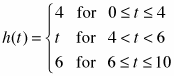, complete the following problems. Homework Help ✎

1. Graph h(t) over the interval 0 ≤ t ≤ 10.

2. What is the area under h(t) over the interval ≤ t ≤ 10?

3. If g(t) = h(t) + 6, what is the area under g(t) for the same interval?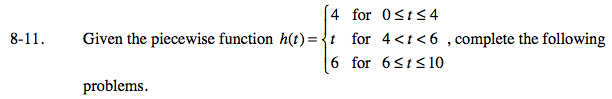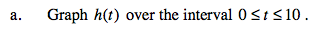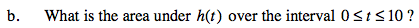Break the area into smaller rectangles and triangles. Calculate the area for each. Add up the areas of the parts.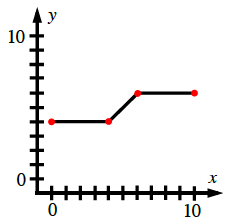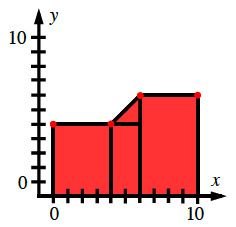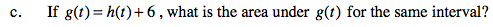Raising the graph up 6 would give the area an additional (6)(width).